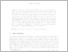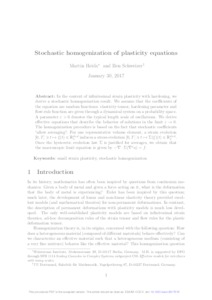Repository: Freie Universität Berlin, Math Department

# Stochastic homogenization of plasticity equations

Heida, M. and Schweizer, B. (2017) Stochastic homogenization of plasticity equations. ESAIM: Control, Optimisation and Calculus of Variations . pp. 1-30. (Submitted)Preview
PDF - Accepted Version
519kB

Official URL: https://dx.doi.org/10.1051/cocv/2017015

## Abstract

In the context of infinitesimal strain plasticity with hardening, we derive a stochastic homogenization result. We assume that the coefficients of the equation are random functions: elasticity tensor, hardening parameter and flow-rule function are given through a dynamical system on a probability space. A parameter \epsilon > 0 denotes the typical length scale of oscillations. We derive effective equations that describe the behavior of solutions in the limit \epsion -> 0. The homogenization procedure is based on the fact that stochastic coefficients “allow averaging”: For one representative volume element, a strain evolution [0; T] \ni t \mapsto\xi(t) \in Rd^dxd _s induces a stress evolution [0; T] \ni t \mapsto\Sigma(\xi)(t) \in Rd^dxd _s . Once the hysteretic evolution law \Sigma is justified for averages, we obtain that the macroscopic limit equation is given by -\triangledown\cdot\Sigma(\triangledown^s u) = f.

Item Type: Article SFB 1114 Preprint 04/2017 in arXiv:1604.02291 Mathematical and Computer Sciences > Mathematics > Numerical Analysis Department of Mathematics and Computer Science > Institute of Mathematics 2151 Silvia Hoemke 07 Dec 2017 17:29 08 Dec 2017 14:00

Repository Staff Only: item control page